### Home > A2C > Chapter 3 > Lesson 3.2.3 > Problem3-125

3-125.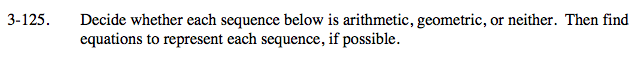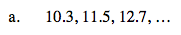Notice that the increase is not a constant number.

The sequence is arithmetic.
t(n) = 9.1 + 1.2n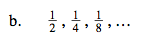Notice the ratio.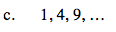Notice that each number is a perfect square.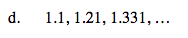Notice that the sequence's increase is constant.

Remember what 11 squared is.

The sequence is geometric.
t(n) = 1.1n